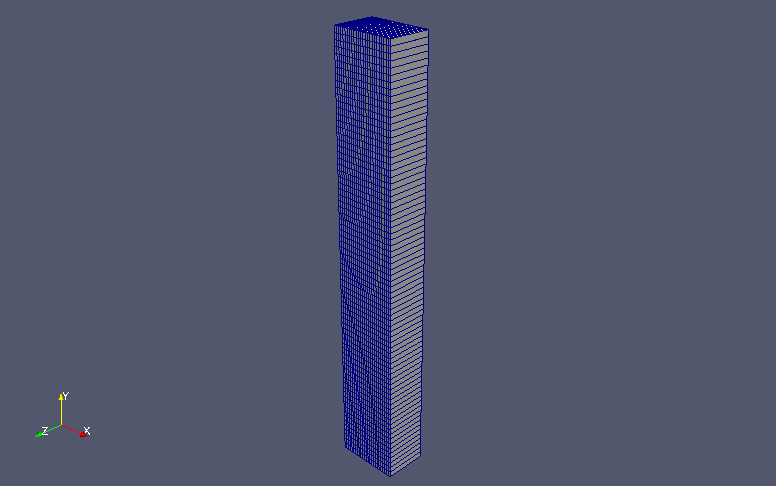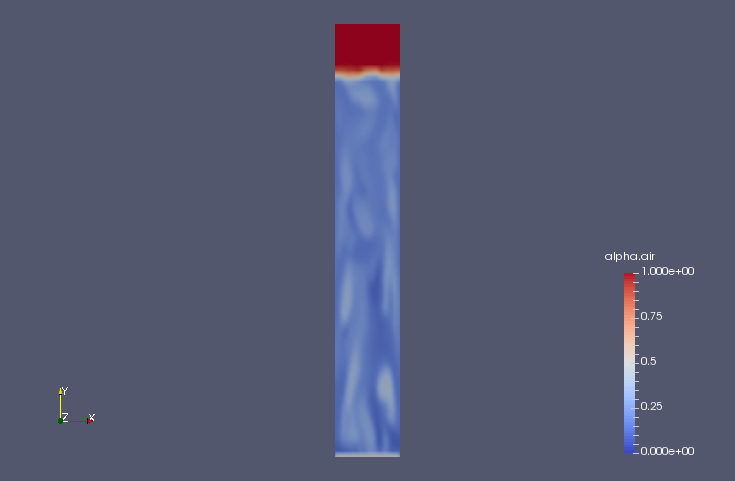﻿ Blowing air into water column (Laminar, IATE model) - XSim

# Blowing air into water column (Laminar, IATE model)

OpenFOAM 4.x

## Case directory

\$FOAM_TUTORIALS/multiphase/twoPhaseEulerFoam/laminar/bubbleColumnIATE

## SummaryModel geometry

We calculate the mixing of air into the water column for 100 seconds. Air flows in from the region "inlet" at the bottom of the water column at (0, 0.1, 0) m/s and out from the region "outlet". We assume that the ratio of water to air is 1:1 in the region "inlet", and the calculation is performed as a two-dimensional problem with one mesh in the z-direction.

The properties of the air and water are set in the files "constant/phaseProperties", "constant/thermophysicalProperties.air", and "constant/thermophysicalProperties.water".

The bubble diameter is calculated based on the IATE (Interfacial Area Transport Equation) model. Each parameter of the model is specified in the file "constant/phaseProperties" as follows.

```air
{
diameterModel   IATE;
IATECoeffs
{
dMax 1e-2;
dMin 1e-4;
residualAlpha 1e-6;
sources
(
wakeEntrainmentCoalescence
{
Cwe         0.002;
}
randomCoalescence
{
Crc         0.04;
C           3;
alphaMax    0.75;
}
turbulentBreakUp
{
Cti         0.085;
WeCr        6;
}
);
}
residualAlpha   1e-6;
}
```

The meshes are as follows, and the number of mesh is 1875.Meshes

The calculation result is as follows.

Volume ratio of air (alpha.air)Volume ratio of air at final time (alpha.air)

## Commands

cp -r \$FOAM_TUTORIALS/multiphase/twoPhaseEulerFoam/laminar/bubbleColumnIATE bubbleColumnIATE
cd bubbleColumnIATE

blockMesh

setFields
twoPhaseEulerFoam

paraFoam

## Calculation time

15 minutes 28.42 seconds *Single, Inter(R) Core(TM) i7-2600 CPU @ 3.40GHz 3.40GHz